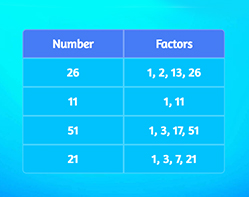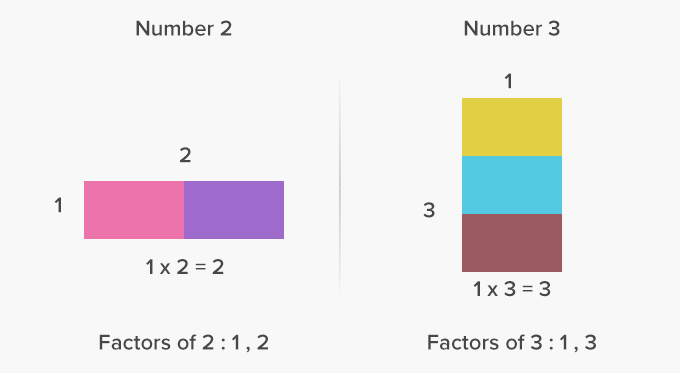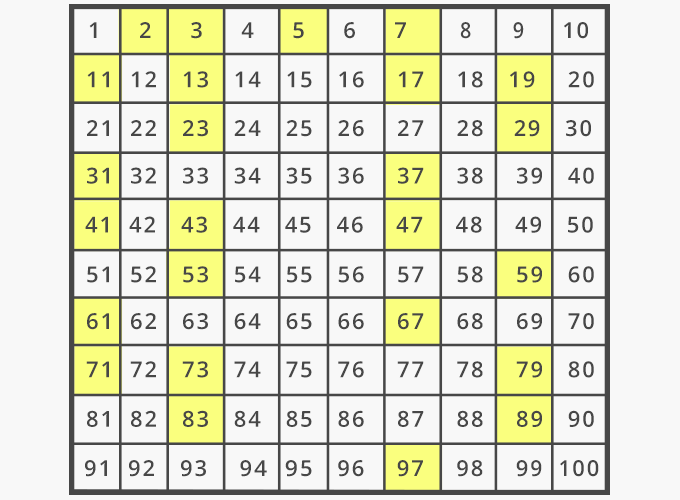# Prime Number - Definition with Examples

The Complete K-5 Math Learning Program Built for Your Child

• 30 Million Kids

Loved by kids and parent worldwide

• 50,000 Schools

Trusted by teachers across schools

• Comprehensive Curriculum

Aligned to Common Core

## Prime Number GamesPrime and Composite Numbers

Identify prime and composite numbers. A prime number has only 2 factors, the number 1 and itself. Remember, that 1 is neither prime nor composite.

Covers Common Core Curriculum 4.OA.4

## What are Prime Numbers?

In math, prime numbers are whole numbers greater than 1, that have only two factors – 1 and the number itself.
Prime numbers are divisible only by the number 1 or itself.

For example, 2, 3, 5, 7 and 11 are the first few prime numbers.Here’s a list of all the prime numbers up to 100, marked in yellow.Fun Facts 1 is neither a prime number nor a composite number. The only even prime number is 2. No prime number greater than 5  ends in a 5.

##Let's sing!

Prime numbers are 2, 3, 5 and 7,
And so is the whole number 11.
They have no other factors on the shelf,
Except for 1 and the number itself!

##Let's do it!

Ask your children to identify the numbers you say as prime or not prime. You can further ask them to find the prime number factors that the ‘not prime’ numbers consist of.

##Related math vocabulary

Won Numerous Awards & Honors